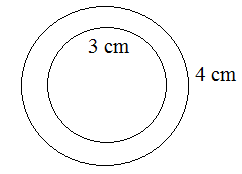# Ex.11.3 Q5 Perimeter and Area - NCERT Maths Class 7

## Question

From a circular sheet of radius $$4 \rm\,cm$$, a circle of radius $$3\rm\, cm$$ is removed. Find the area of the remaining sheet. \begin{align}(\text{Take}\, π = 3.14)\end{align}

Video Solution
Perimeter And Area
Ex 11.3 | Question 5

## Text Solution

What is known?

Radius of circular sheet from which a circle of radius $$3\rm\, cm$$ is removed.

What is the unknown?

The area of the remaining sheet.

Reasoning:

To find the area of the remaining sheet, first find the area of both the circular sheets and then subtract the area of circle sheet of radius $$3\rm\, cm$$ from the area of circular sheet of radius $$4 \rm\,cm$$.Steps:

Radius of circular sheet (r1) $$=$$ $$4 \rm\,cm$$

Radius of removed circle (r2) $$=$$ $$3\rm\, cm$$

Area of remaining sheet $$=$$ Area of circular sheet $$-$$ Area of removed circle

\begin{align} &= \pi {r_1}^2 - \pi {r_2}^2\\ &= \pi \left( {{r_1}^2 - {r_2}^2} \right)\\ &= 3.14{\rm{ }}\left[ {\left( {{4^2}} \right) - \left( {{3^2}} \right)} \right]\\ &= 3.14\left( {16 - 9} \right)\\ &= 3.14 \times 7\\ &= 21.98{\rm{ }}\rm\,c{m^2}\end{align}

Thus, the area of remaining sheet is $$21.98 \rm\,cm^2.$$

Learn from the best math teachers and top your exams

• Live one on one classroom and doubt clearing
• Practice worksheets in and after class for conceptual clarity
• Personalized curriculum to keep up with school